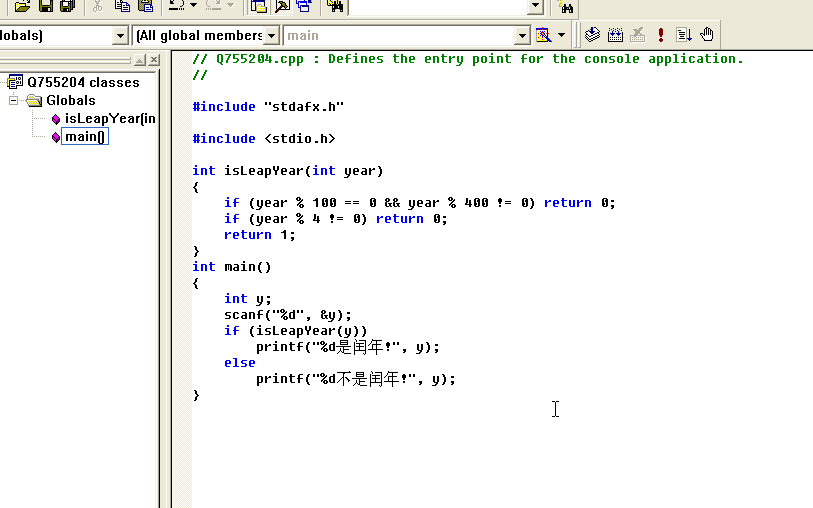8个回答

``````#include <stdio.h>

int isLeapYear(int year)
{
if (year % 100 == 0 && year % 400 != 0) return 0;
if (year % 4 != 0) return 0;
return 1;
}
int main()
{
int y;
scanf("%d", &y);
if (isLeapYear(y))
printf("%dÊÇÈòÄê!", y);
else
printf("%d²»ÊÇÈòÄê!", y);
}
``````bool isRunYear(int year)
{
return ((year % 4 == 0 && year % 100 != 0) || year % 400 == 0) ? 1 : 0;
}

bool isRunYear(int year)
{
return ((year % 4 == 0 && year % 100 != 0) || year % 400 == 0);
}

int isRunYear(int year)
{
return ((year % 4 == 0 && year % 100 != 0) || year % 400 == 0) ? 1 : 0;
}

#include
int main()
{
int year,leap;
scanf("%d",&year);
if(year%4==0)
{
if(year%100!=0)
leap=1;
if(year%400==0)
leap=1;
}
if(leap==1)
printf("yes");
else
printf("no");
return 0;
}

#include
int LeapYear(int year);

int main()
{
int year;
scanf("%d",&year);
if(LeapYear(year)==0)
printf("%d是闰年\n",year);
else
printf("%d不是闰年\n",year);
return 0;
}

int LeapYear(int year)
{
if(year%100 == 0&&year%400 == 0)
return 0;
else if(year%4 == 0)
return 0;
else
return 1;
}

#include
int isLeapYear(int year)
{
if (year % 4 == 0 && year % 100 != 0) return 1;
if (year %400== 0) return 1;
return 0;
}
int main()
{
int y;
scanf("%d", &y);
if (isLeapYear(y))
printf("%d is a leapyear", y);
else
printf("%d is not a leapyear", y);
return 0;
}

``````#include <stdio.h>
int main()
{
int year,i;
printf("请输人年份：\n");
scanf("%d",&year);

if(year%400==0)
i=1;
else
{
if(year%4==0&&year%100!=0)
i=1;
else
i=0;
}
if(i==1)
{
printf("%d 年是闰年\n",year);
}
else
{
printf("%d 年非闰年\n",year);
}
return 0;
}
``````